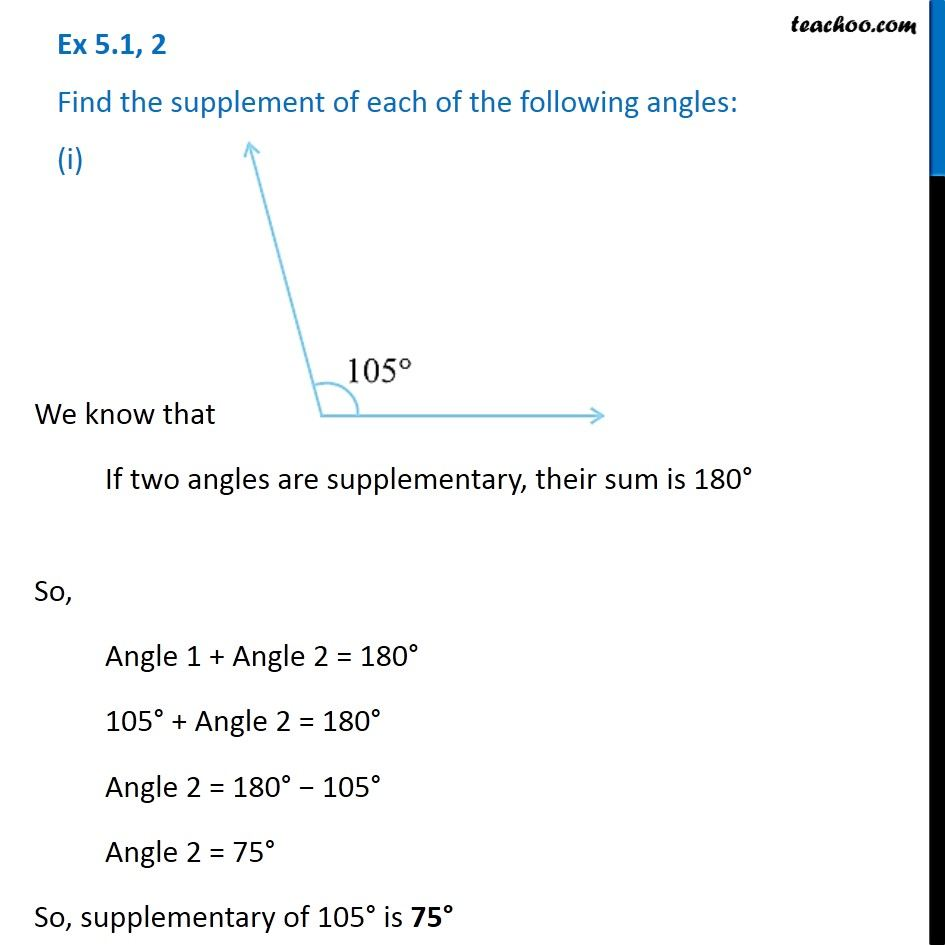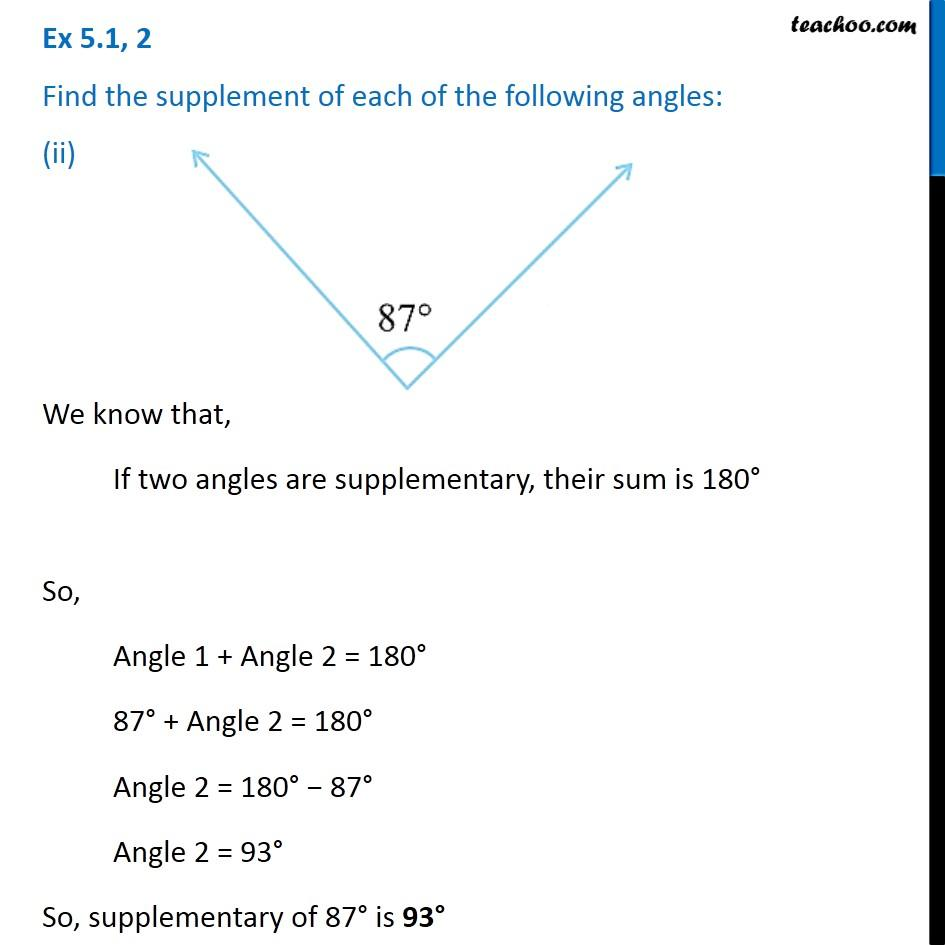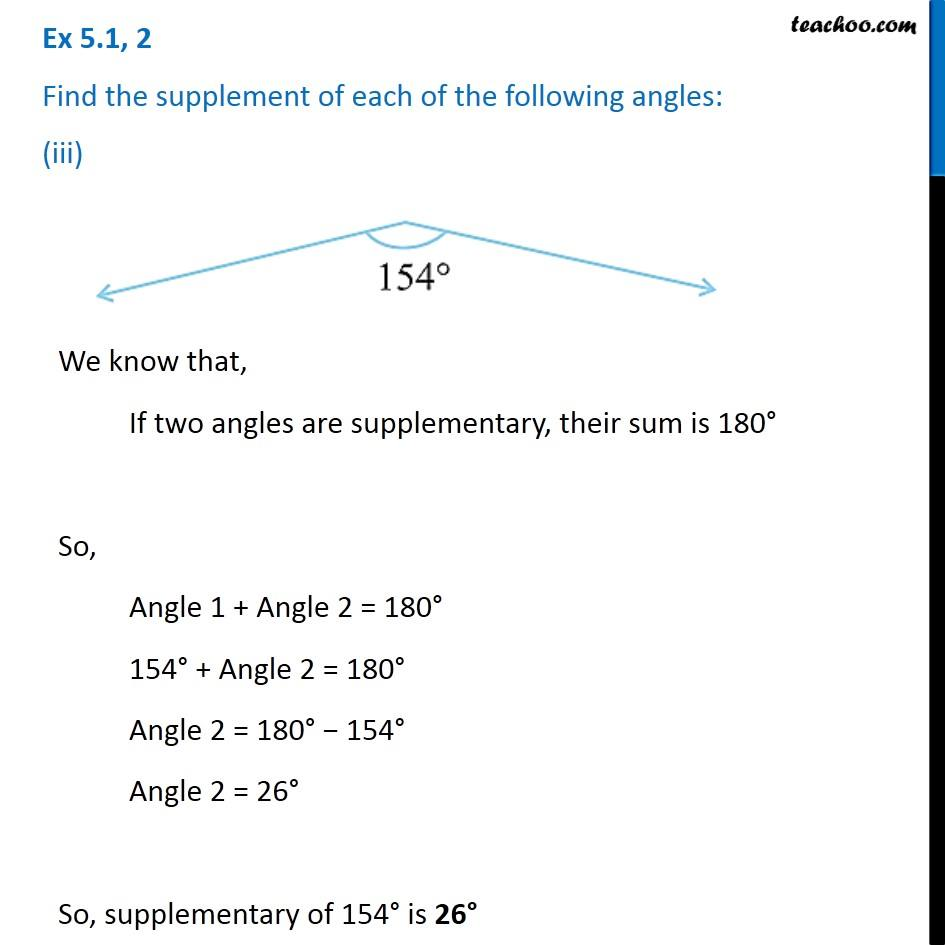1. Chapter 5 Class 7 Lines and Angles
2. Concept wise
3. Supplementary and complementary angles

Transcript

Ex 5.1, 2 Find the supplement of each of the following angles: (i)We know that, If two angles are supplementary, their sum is 180° So, Angle 1 + Angle 2 = 180° 105° + Angle 2 = 180° Angle 2 = 180° − 105° Angle 2 = 75° So, supplementary of 105° is 75° Ex 5.1, 2 Find the supplement of each of the following angles: (ii) We know that, If two angles are supplementary, their sum is 180° So, Angle 1 + Angle 2 = 180° 87° + Angle 2 = 180° Angle 2 = 180° − 87° Angle 2 = 93° So, supplementary of 87° is 93° Ex 5.1, 2 Find the supplement of each of the following angles: (iii) We know that, If two angles are supplementary, their sum is 180° So, Angle 1 + Angle 2 = 180° 154° + Angle 2 = 180° Angle 2 = 180° − 154° Angle 2 = 26° So, supplementary of 154° is 26°

Supplementary and complementary angles